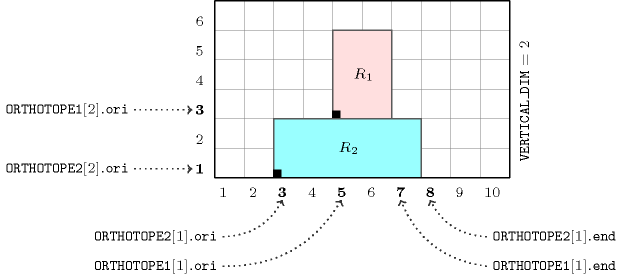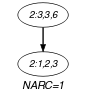## 5.312. orth_on_top_of_orth

Origin
Constraint

$\mathrm{𝚘𝚛𝚝𝚑}_\mathrm{𝚘𝚗}_\mathrm{𝚝𝚘𝚙}_\mathrm{𝚘𝚏}_\mathrm{𝚘𝚛𝚝𝚑}\left(\mathrm{𝙾𝚁𝚃𝙷𝙾𝚃𝙾𝙿𝙴}\mathtt{1},\mathrm{𝙾𝚁𝚃𝙷𝙾𝚃𝙾𝙿𝙴}\mathtt{2},\mathrm{𝚅𝙴𝚁𝚃𝙸𝙲𝙰𝙻}_\mathrm{𝙳𝙸𝙼}\right)$

Type
 $\mathrm{𝙾𝚁𝚃𝙷𝙾𝚃𝙾𝙿𝙴}$ $\mathrm{𝚌𝚘𝚕𝚕𝚎𝚌𝚝𝚒𝚘𝚗}\left(\mathrm{𝚘𝚛𝚒}-\mathrm{𝚍𝚟𝚊𝚛},\mathrm{𝚜𝚒𝚣}-\mathrm{𝚍𝚟𝚊𝚛},\mathrm{𝚎𝚗𝚍}-\mathrm{𝚍𝚟𝚊𝚛}\right)$
Arguments
 $\mathrm{𝙾𝚁𝚃𝙷𝙾𝚃𝙾𝙿𝙴}\mathtt{1}$ $\mathrm{𝙾𝚁𝚃𝙷𝙾𝚃𝙾𝙿𝙴}$ $\mathrm{𝙾𝚁𝚃𝙷𝙾𝚃𝙾𝙿𝙴}\mathtt{2}$ $\mathrm{𝙾𝚁𝚃𝙷𝙾𝚃𝙾𝙿𝙴}$ $\mathrm{𝚅𝙴𝚁𝚃𝙸𝙲𝙰𝙻}_\mathrm{𝙳𝙸𝙼}$ $\mathrm{𝚒𝚗𝚝}$
Restrictions
 $|\mathrm{𝙾𝚁𝚃𝙷𝙾𝚃𝙾𝙿𝙴}|>0$ $\mathrm{𝚛𝚎𝚚𝚞𝚒𝚛𝚎}_\mathrm{𝚊𝚝}_\mathrm{𝚕𝚎𝚊𝚜𝚝}$$\left(2,\mathrm{𝙾𝚁𝚃𝙷𝙾𝚃𝙾𝙿𝙴},\left[\mathrm{𝚘𝚛𝚒},\mathrm{𝚜𝚒𝚣},\mathrm{𝚎𝚗𝚍}\right]\right)$ $\mathrm{𝙾𝚁𝚃𝙷𝙾𝚃𝙾𝙿𝙴}.\mathrm{𝚜𝚒𝚣}\ge 0$ $\mathrm{𝙾𝚁𝚃𝙷𝙾𝚃𝙾𝙿𝙴}.\mathrm{𝚘𝚛𝚒}\le \mathrm{𝙾𝚁𝚃𝙷𝙾𝚃𝙾𝙿𝙴}.\mathrm{𝚎𝚗𝚍}$ $|\mathrm{𝙾𝚁𝚃𝙷𝙾𝚃𝙾𝙿𝙴}\mathtt{1}|=|\mathrm{𝙾𝚁𝚃𝙷𝙾𝚃𝙾𝙿𝙴}\mathtt{2}|$ $\mathrm{𝚅𝙴𝚁𝚃𝙸𝙲𝙰𝙻}_\mathrm{𝙳𝙸𝙼}\ge 1$ $\mathrm{𝚅𝙴𝚁𝚃𝙸𝙲𝙰𝙻}_\mathrm{𝙳𝙸𝙼}\le |\mathrm{𝙾𝚁𝚃𝙷𝙾𝚃𝙾𝙿𝙴}\mathtt{1}|$ $\mathrm{𝚘𝚛𝚝𝚑}_\mathrm{𝚕𝚒𝚗𝚔}_\mathrm{𝚘𝚛𝚒}_\mathrm{𝚜𝚒𝚣}_\mathrm{𝚎𝚗𝚍}$$\left(\mathrm{𝙾𝚁𝚃𝙷𝙾𝚃𝙾𝙿𝙴}\mathtt{1}\right)$ $\mathrm{𝚘𝚛𝚝𝚑}_\mathrm{𝚕𝚒𝚗𝚔}_\mathrm{𝚘𝚛𝚒}_\mathrm{𝚜𝚒𝚣}_\mathrm{𝚎𝚗𝚍}$$\left(\mathrm{𝙾𝚁𝚃𝙷𝙾𝚃𝙾𝙿𝙴}\mathtt{2}\right)$
Purpose

$\mathrm{𝙾𝚁𝚃𝙷𝙾𝚃𝙾𝙿𝙴}\mathtt{1}$ is located on top of $\mathrm{𝙾𝚁𝚃𝙷𝙾𝚃𝙾𝙿𝙴}\mathtt{2}$ which concretely means:

• In each dimension different from $\mathrm{𝚅𝙴𝚁𝚃𝙸𝙲𝙰𝙻}_\mathrm{𝙳𝙸𝙼}$ the projection of $\mathrm{𝙾𝚁𝚃𝙷𝙾𝚃𝙾𝙿𝙴}\mathtt{1}$ is included in the projection of $\mathrm{𝙾𝚁𝚃𝙷𝙾𝚃𝙾𝙿𝙴}\mathtt{2}$.

• In the dimension $\mathrm{𝚅𝙴𝚁𝚃𝙸𝙲𝙰𝙻}_\mathrm{𝙳𝙸𝙼}$ the origin of $\mathrm{𝙾𝚁𝚃𝙷𝙾𝚃𝙾𝙿𝙴}\mathtt{1}$ coincide with the end of $\mathrm{𝙾𝚁𝚃𝙷𝙾𝚃𝙾𝙿𝙴}\mathtt{2}$.

Example
$\left(\begin{array}{c}〈\mathrm{𝚘𝚛𝚒}-5\mathrm{𝚜𝚒𝚣}-2\mathrm{𝚎𝚗𝚍}-7,\mathrm{𝚘𝚛𝚒}-3\mathrm{𝚜𝚒𝚣}-3\mathrm{𝚎𝚗𝚍}-6〉,\hfill \\ 〈\mathrm{𝚘𝚛𝚒}-3\mathrm{𝚜𝚒𝚣}-5\mathrm{𝚎𝚗𝚍}-8,\mathrm{𝚘𝚛𝚒}-1\mathrm{𝚜𝚒𝚣}-2\mathrm{𝚎𝚗𝚍}-3〉,2\hfill \end{array}\right)$

As illustrated by Figure 5.312.1 the orthotope $\mathrm{𝙾𝚁𝚃𝙷𝙾𝚃𝙾𝙿𝙴}\mathtt{1}$ (rectangle R1 coloured in pink) is on top of $\mathrm{𝙾𝚁𝚃𝙷𝙾𝚃𝙾𝙿𝙴}\mathtt{2}$ (rectangle R2 coloured in blue) according to the hypothesis that the vertical dimension corresponds to dimension 2 (i.e., $\mathrm{𝚅𝙴𝚁𝚃𝙸𝙲𝙰𝙻}_\mathrm{𝙳𝙸𝙼}=2$). This stands from the fact that the following conditions hold:

• $\mathrm{𝙾𝚁𝚃𝙷𝙾𝚃𝙾𝙿𝙴}\mathtt{2}\left[2\right].\mathrm{𝚘𝚛𝚒}+\mathrm{𝙾𝚁𝚃𝙷𝙾𝚃𝙾𝙿𝙴}\mathtt{2}\left[2\right].\mathrm{𝚜𝚒𝚣}=1+2=\mathrm{𝙾𝚁𝚃𝙷𝙾𝚃𝙾𝙿𝙴}\mathtt{1}\left[2\right].\mathrm{𝚘𝚛𝚒}$,

• $\mathrm{𝙾𝚁𝚃𝙷𝙾𝚃𝙾𝙿𝙴}\mathtt{2}\left[1\right].\mathrm{𝚘𝚛𝚒}=3\le \mathrm{𝙾𝚁𝚃𝙷𝙾𝚃𝙾𝙿𝙴}\mathtt{1}\left[1\right].\mathrm{𝚘𝚛𝚒}=5$,

• $\mathrm{𝙾𝚁𝚃𝙷𝙾𝚃𝙾𝙿𝙴}\mathtt{1}\left[1\right].\mathrm{𝚎𝚗𝚍}=7\le \mathrm{𝙾𝚁𝚃𝙷𝙾𝚃𝙾𝙿𝙴}\mathtt{2}\left[1\right].\mathrm{𝚎𝚗𝚍}=8$.

Consequently, the $\mathrm{𝚘𝚛𝚝𝚑}_\mathrm{𝚘𝚗}_\mathrm{𝚝𝚘𝚙}_\mathrm{𝚘𝚏}_\mathrm{𝚘𝚛𝚝𝚑}$ constraint holds.

##### Figure 5.312.1. Illustration of the relation on top of of the Example slot (${R}_{1}$ on top of ${R}_{2}$ wrt dimension $\mathrm{𝚅𝙴𝚁𝚃𝙸𝙲𝙰𝙻}_\mathrm{𝙳𝙸𝙼}=2$)Typical
 $|\mathrm{𝙾𝚁𝚃𝙷𝙾𝚃𝙾𝙿𝙴}|>1$ $\mathrm{𝙾𝚁𝚃𝙷𝙾𝚃𝙾𝙿𝙴}.\mathrm{𝚜𝚒𝚣}>0$
Used in
Keywords
Arc input(s)

$\mathrm{𝙾𝚁𝚃𝙷𝙾𝚃𝙾𝙿𝙴}\mathtt{1}$ $\mathrm{𝙾𝚁𝚃𝙷𝙾𝚃𝙾𝙿𝙴}\mathtt{2}$

Arc generator
$\mathrm{𝑃𝑅𝑂𝐷𝑈𝐶𝑇}$$\left(=\right)↦\mathrm{𝚌𝚘𝚕𝚕𝚎𝚌𝚝𝚒𝚘𝚗}\left(\mathrm{𝚘𝚛𝚝𝚑𝚘𝚝𝚘𝚙𝚎}\mathtt{1},\mathrm{𝚘𝚛𝚝𝚑𝚘𝚝𝚘𝚙𝚎}\mathtt{2}\right)$

Arc arity
Arc constraint(s)
 $•\mathrm{𝚘𝚛𝚝𝚑𝚘𝚝𝚘𝚙𝚎}\mathtt{1}.\mathrm{𝚔𝚎𝚢}\ne \mathrm{𝚅𝙴𝚁𝚃𝙸𝙲𝙰𝙻}_\mathrm{𝙳𝙸𝙼}$ $•\mathrm{𝚘𝚛𝚝𝚑𝚘𝚝𝚘𝚙𝚎}\mathtt{2}.\mathrm{𝚘𝚛𝚒}\le \mathrm{𝚘𝚛𝚝𝚑𝚘𝚝𝚘𝚙𝚎}\mathtt{1}.\mathrm{𝚘𝚛𝚒}$ $•\mathrm{𝚘𝚛𝚝𝚑𝚘𝚝𝚘𝚙𝚎}\mathtt{1}.\mathrm{𝚎𝚗𝚍}\le \mathrm{𝚘𝚛𝚝𝚑𝚘𝚝𝚘𝚙𝚎}\mathtt{2}.\mathrm{𝚎𝚗𝚍}$
Graph property(ies)
$\mathrm{𝐍𝐀𝐑𝐂}$$=|\mathrm{𝙾𝚁𝚃𝙷𝙾𝚃𝙾𝙿𝙴}\mathtt{1}|-1$

Arc input(s)

$\mathrm{𝙾𝚁𝚃𝙷𝙾𝚃𝙾𝙿𝙴}\mathtt{1}$ $\mathrm{𝙾𝚁𝚃𝙷𝙾𝚃𝙾𝙿𝙴}\mathtt{2}$

Arc generator
$\mathrm{𝑃𝑅𝑂𝐷𝑈𝐶𝑇}$$\left(=\right)↦\mathrm{𝚌𝚘𝚕𝚕𝚎𝚌𝚝𝚒𝚘𝚗}\left(\mathrm{𝚘𝚛𝚝𝚑𝚘𝚝𝚘𝚙𝚎}\mathtt{1},\mathrm{𝚘𝚛𝚝𝚑𝚘𝚝𝚘𝚙𝚎}\mathtt{2}\right)$

Arc arity
Arc constraint(s)
 $•\mathrm{𝚘𝚛𝚝𝚑𝚘𝚝𝚘𝚙𝚎}\mathtt{1}.\mathrm{𝚔𝚎𝚢}=\mathrm{𝚅𝙴𝚁𝚃𝙸𝙲𝙰𝙻}_\mathrm{𝙳𝙸𝙼}$ $•\mathrm{𝚘𝚛𝚝𝚑𝚘𝚝𝚘𝚙𝚎}\mathtt{1}.\mathrm{𝚘𝚛𝚒}=\mathrm{𝚘𝚛𝚝𝚑𝚘𝚝𝚘𝚙𝚎}\mathtt{2}.\mathrm{𝚎𝚗𝚍}$
Graph property(ies)
$\mathrm{𝐍𝐀𝐑𝐂}$$=1$

Graph model

The first and second graph constraints respectively express the first and second conditions stated in the Purpose slot defining the $\mathrm{𝚘𝚛𝚝𝚑}_\mathrm{𝚘𝚗}_\mathrm{𝚝𝚘𝚙}_\mathrm{𝚘𝚏}_\mathrm{𝚘𝚛𝚝𝚑}$ constraint.

Parts (A) and (B) of Figure 5.312.2 respectively show the initial and final graph associated with the second graph constraint of the Example slot. Since we use the $\mathrm{𝐍𝐀𝐑𝐂}$ graph property, the unique arc of the final graph is stressed in bold.

##### Figure 5.312.2. Initial and final graph of the $\mathrm{𝚘𝚛𝚝𝚑}_\mathrm{𝚘𝚗}_\mathrm{𝚝𝚘𝚙}_\mathrm{𝚘𝚏}_\mathrm{𝚘𝚛𝚝𝚑}$ constraint(a) (b)
Signature

Consider the second graph constraint. Since all the $\mathrm{𝚔𝚎𝚢}$ attributes of the $\mathrm{𝙾𝚁𝚃𝙷𝙾𝚃𝙾𝙿𝙴}\mathtt{1}$ collection are distinct, because of the arc constraint $\mathrm{𝚘𝚛𝚝𝚑𝚘𝚝𝚘𝚙𝚎}\mathtt{1}.\mathrm{𝚔𝚎𝚢}=\mathrm{𝚅𝙴𝚁𝚃𝙸𝙲𝙰𝙻}_\mathrm{𝙳𝙸𝙼}$, and since we use the $\mathrm{𝑃𝑅𝑂𝐷𝑈𝐶𝑇}\left(=\right)$ arc generator the final graph contains at most one arc. Therefore we can rewrite the graph property $\mathrm{𝐍𝐀𝐑𝐂}=1$ to $\mathrm{𝐍𝐀𝐑𝐂}\ge 1$ and simplify $\underline{\overline{\mathrm{𝐍𝐀𝐑𝐂}}}$ to $\overline{\mathrm{𝐍𝐀𝐑𝐂}}$.